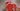# 传统色谱，中文颜色名称(chinese color names)

## 红色系颜色名称

#ffb3a7
RGB(255, 179, 167)

#ed5736
RGB(237, 87, 54)

#f00056
RGB(240, 0, 86)

#f47983
RGB(244, 121, 131)

#db5a6b
RGB(219, 90, 107)

#f20c00
RGB(242, 12, 0)

#c93756
RGB(201, 55, 86)

#f05654
RGB(240, 86, 84)

#ff2121
RGB(255, 33, 33)

#8c4356
RGB(140, 67, 86)

#c83c23
RGB(200, 60, 35)

#9d2933
RGB(157, 41, 51)

#ff4c00
RGB(255, 76, 0)

#ff4e20
RGB(255, 78, 32)

#f35336
RGB(243, 83, 54)

#cb3a56
RGB(203, 58, 86)

#ff2d51
RGB(255, 45, 81)

#c91f37
RGB(201, 31, 55)

#ef7a82
RGB(239, 122, 130)

#ff0097
RGB(255, 0, 151)

#ff3300
RGB(255, 51, 0)

#c3272b
RGB(195, 39, 43)

#a98175
RGB(169, 129, 117

#c32136
RGB(179, 109, 97)

#b36d61
RGB(179, 109, 97)

#be002f
RGB(190, 0, 47)

#dc3023
RGB(220, 48, 35)

#f9906f
RGB(249, 144, 111)

## 黄色系颜色名称

#fff143
RGB(255, 241, 67)

#faff72
RGB(250, 255, 114)

#eaff56
RGB(234, 255, 86)

#ffa631
RGB(255, 166, 49)

#ff8c31
RGB(255, 140, 49)

#ff8936
RGB(255, 137, 54)

#ffa400
RGB(255, 164, 0)

#ff7500
RGB(255, 117, 0)

#ffc773
RGB(255, 199, 115)

#f0c239
RGB(240, 194, 57)

#fa8c35
RGB(250, 140, 53)

#b35c44
RGB(179, 92, 68)

#a88462
RGB(168, 132, 98)

#c89b40
RGB(200, 155, 64)

#60281e
RGB(96, 40, 30)

#b25d25
RGB(178, 93, 37)

#827100
RGB(130, 113, 0)

#7c4b00
RGB(124, 75, 0)

#9b4400
RGB(155, 68, 0)

#ae7000
RGB(174, 112, 0)

#9c5333
RGB(156, 83, 51)

#955539
RGB(149, 85, 57)

#ca6924
RGB(202, 105, 36)

#6e511e
RGB(110, 81, 30)

#d3b17d
RGB(211, 177, 125)

#e29c45
RGB(226, 156, 69)

#896c39
RGB(137, 108, 57)

#d9b611
RGB(217, 182, 17)

## 绿色系颜色名称

#bddd22
RGB(189, 221, 34)

#c9dd22
RGB(201, 221, 34)

#afdd22
RGB(175, 221, 34)

#789262
RGB(120, 146, 98)

#a3d900
RGB(163, 217, 0)

#9ed900
RGB(158, 217, 0)

#0eb83a
RGB(14, 184, 58)

#0eb83a
RGB(14, 184, 58)

#0aa344
RGB(10, 163, 68)

#00bc12
RGB(0, 188, 18)

#0c8918
RGB(12, 137, 24)

#1bd1a5
RGB(27, 209, 165)

RGB(42, 221, 156)

#48c0a3
RGB(72, 192, 163)

RGB(61, 225, 173)

#40de5a
RGB(64, 222, 90)

#00e09e
RGB(0, 224, 158)

#00e079
RGB(0, 224, 121)

#c0ebd7
RGB(192, 235, 215)

#e0eee8
RGB(224, 238, 232)

#bbcdc5
RGB(187, 205, 197)

#424c50
RGB(66, 76, 80)

#00e500
RGB(0, 229, 0)

#9ed048
RGB(158, 208, 72)

#96ce54
RGB(150, 206, 84)

#7bcfa6
RGB(123, 207, 166)

#2edfa3
RGB(46, 223, 163)

RGB(127, 236, 173)

#a4e2c6
RGB(164, 226, 198)

#21a675
RGB(33, 166, 117)

#057748
RGB(5, 119, 72)

#bce672
RGB(188, 230, 114)

## 蓝色系颜色名称

#44cef6
RGB(68, 206, 246)

#177cb0
RGB(23, 124, 176)

#065279
RGB(6, 82, 121)

#3eede7
RGB(62, 237, 231)

#70f3ff
RGB(112, 243, 255)

#4b5cc4
RGB(75, 92, 196)

#a1afc9
RGB(161, 175, 201)

#2e4e7e
RGB(46, 78, 126)

#3b2e7e
RGB(59, 46, 126)

#4a4266
RGB(74, 66, 102)

#4a4266
RGB(74, 66, 102)

#4a4266
RGB(74, 66, 102)

#426666
RGB(66, 102, 102)

#425066
RGB(66, 80, 102)

#574266
RGB(87, 66, 102)

#8d4bbb
RGB(141, 75, 187)

#815463
RGB(129, 84, 99)

#815476
RGB(129, 84, 118)

#4c221b
RGB(76, 34, 27)

#003371
RGB(0, 51, 113)

#56004f
RGB(86, 0, 79)

#801dae
RGB(128, 29, 174)

#4c8dae
RGB(76, 141, 174)

#b0a4e3
RGB(176, 164, 227)

#cca4e3
RGB(204, 164, 227)

#edd1d8
RGB(237, 209, 216)

#e4c6d0
RGB(228, 198, 208)

## 苍色系颜色名称

#75878a
RGB(117, 135, 138)

#519a73
RGB(81, 154, 115)

#a29b7c
RGB(162, 155, 124)

#7397ab
RGB(115, 151, 171)

#395260
RGB(57, 82, 96)

#d1d9e0
RGB(209, 217, 224)

## 水色系颜色名称

RGB(136, 173, 166)

#f3d3e7
RGB(243, 211, 231)

#d4f2e7
RGB(212, 242, 231)

#d2f0f4
RGB(210, 240, 244)

#d3e0f3
RGB(211, 224, 243)

#30dff3
RGB(48, 223, 243)

#25f8cb
RGB(37, 248, 203)

## 灰白色系颜色名称

#ffffff
RGB(255, 255, 255)

#fffbf0
RGB(255, 251, 240)

#f2fdff
RGB(240, 252, 255)

#d6ecf0
RGB(214, 236, 240)

#f2ecde
RGB(242, 236, 222)

#e0f0e9
RGB(224, 240, 233)

#f3f9f1
RGB(243, 249, 241)

#e9f1f6
RGB(233, 241, 246)

#c2ccd0
RGB(194, 204, 208)

#fcefe8
RGB(252, 239, 232)

#e3f9fd
RGB(227, 249, 253)

#808080
RGB(128, 128, 128)

#eedeb0
RGB(238, 222, 176)

#f0f0f4
RGB(240, 240, 244)

## 黑色系颜色名称

#622a1d
RGB(98, 42, 29)

#3d3b4f
RGB(61, 59, 79)

#725e82
RGB(114, 94, 130)

#392f41
RGB(57, 47, 65)

#161823
RGB(22, 24, 35)

#50616d
RGB(80, 97, 109)

#758a99
RGB(117, 138, 153)

#000000
RGB(0, 0, 0)

#493131
RGB(73, 49, 49)

#312520
RGB(49, 37, 32)

#5d513c
RGB(93, 81, 60)

#75664d
RGB(117, 102, 77)

#6b6882
RGB(107, 104, 130)

#665757
RGB(102, 87, 87)

#41555d
RGB(65, 85, 93)

## 金银色系颜色名称

#f2be45
rgb(242, 190, 69)

#eacd76
rgb(234, 205, 118)

#e9e7ef
rgb(233, 231, 239)

#549688
rgb(84, 150, 136)

#a78e44
rgb(167, 142, 68)

#bacac6
rgb(186, 202, 198)